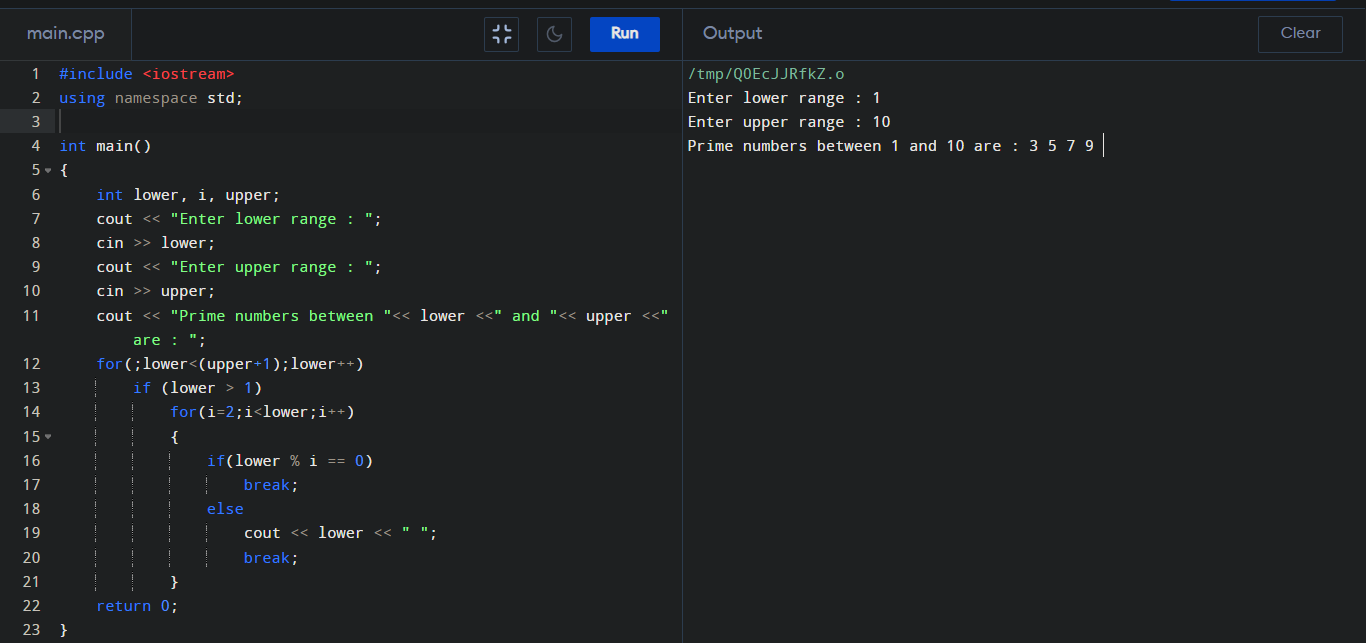C++ Program to print Prime Numbers

To print the prime numbers in a range we need use the following code in C++ program. Here we used 'cout' method to print our desired output.

Prime Numbers in C++ :

#include <iostream>
using namespace std;

int main()
{
int lower, i, upper;
cout << "Enter lower range : ";
cin >> lower;
cout << "Enter upper range : ";
cin >> upper;
cout << "Prime numbers between "<< lower <<" and "<< upper <<" are : ";

for(;lower<(upper+1);lower++)
if (lower > 1)
for(i=2;i<lower;i++)
{
if(lower % i == 0)
break;
else
cout << lower << " ";
break;
}

return 0;
}

Output :